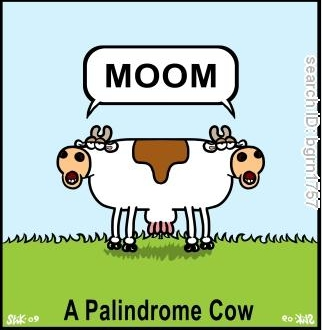# PalPrimesA palindromic-prime or PalPrime is a prime number that is also a palindrome.The first few PalPrimes are $2, 3, 5, 7, 11, 101, 131, 151, 181, 191...$. Let $S$ be the sum of the digits of the largest PalPrime $N$ such that $N<10^9$.What is the value of $S$?

×